# Solid State Important Questions with AnswersSolid State Important Questions with Answers

1. The crystal with metal deficiency defect is
(a) NaCl                      (b) FeO                (c) KCl                        (d) ZnO
Ans 1. (b)

2. Which type of defect has the presence of cations in the interstitials sites?
(a) Schottky defect                             (b) Vacancy defect
(c) Frenkel defect                               (d) Metal deficiency defect
Ans 2. (c)
The defect forms when an atom or smaller ion (usual cation) leaves its place in the lattice, creating a vacancy, and becomes an interstitial by lodging in a nearby location.

3. Which of the following metal oxides is antiferromagnetic in nature?
(a) MnO2                     (b) TiO2                       (c) VO2                      (d) CrO2
Ans 3. (a)
Antiferromagnetism, type of magnetism in solids such as manganese oxide (MnO) in which adjacent ions that behave as tiny magnets (in this case manganese ions, Mn2+) spontaneously align themselves at relatively low temperatures into the opposite, or antiparallel, arrangements throughout the material.

4. Which of the following compound is metallic and ferromagnetic?
(a) CrO2                      (b) VO2                       (c) MnO2                     (d) TiO2
Ans 4. (a)
Only three elements iron (Fe), cobalt (Co), and nickel (Ni) show ferromagnetism at room temperature. CrO2 is also a metallic and ferromagnetic compound that is used to make magnetic tapes for Cassette recorders.

5. Which one of the following is a molecular crystal?
(a) Rock salt               (b) Quartz
(c) Dry ice                  (d) Diamond
Ans 5. (c)
Molecular crystals are substances that have relatively weak intermolecular binding, such as dry ice (solidified carbon dioxide), solid forms of the noble gases (e.g., argon, krypton, and xenon), and crystals of numerous organic compounds.

Solid State Important Questions with Answers

6. Why does ZnO appear golden yellow at high temperatures? Explain?
Ans 6. In ZnO, Zn2+ ions occupy the interstitial sites, and electrons are trapped in the interstitial sites for neutralization. Crystals that show such types of defects are generally colored. Zinc oxide is white in color but on heating, it turns yellow because it loses oxygen reversibly at high temperature.

7. Why is Frenkel defect not found in pure alkali metal halides?
Ans 7. Frenkel defect is not found in the pure alkali metal halides because this defect is due to the vacancy of ion, which is shifted in interstitial spaces, and pure alkyl halides do not have a vacancy.

8. Analysis shows that nickel oxide has the formula Ni.98O1.00. What fractions of the Nickel exist as Ni+2 and Ni+3?
Ans 8. As per the formula, there are 98 Ni ions for 100 oxide ions.
Out of 98 Ni ions, let x ions be in a +2 oxidation state.
98−x ions will be in a +3 oxidation state.
Oxide ion has a −2 charge.
To maintain electrical neutrality, total positive charge on cations = total negative charge on anions.
2x + 3(98−x) + 100(−2) = 0
x=94
Fraction of Ni2+ ion = 94/98 = 0.96
Fraction of Ni3+ ion = (98 – 94)/98 = 0.04

9. Calculate the packing efficiency in a face-centered cubic arrangement?
Ans 9. Packing Efficiency for Face Centred Cubic Unit Cell: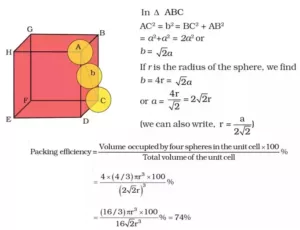Solid State Important Questions with Answers

10. Why does the electrical conductivity of semiconductor increases with rise in temperature?
Ans 10. The gap between the conduction band and valence band is small in semiconductors, therefore, electrons from the valence band can jump to the conduction band on increasing temperature. Thus they become more conducting as the temperature increases.

11. An element with a molar mass of 27g mold forms a cubic unit cell with an edge length of 4.05×10-8 cm. If its density is 2.7 g cm-3, what is the nature of the unit cell?
Ans 11. We Know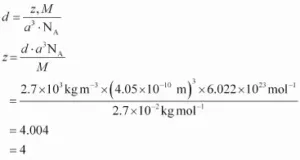12. Why does LiCl acquire pink colour when heated in Li vapours?
Ans 12. On heating LiCl crystals in the presence of an excess Lithium, Clions from crystal diffuse on the surface and combine with ionized Li to form LiCl. The released unpaired electrons occupy the anionic sites known as F centers.
The pink colour results by excitation of these electrons when they absorb energy from visible light falling on them.

13. An element having bcc geometry has an atomic mass of 50u. Calculate the density of the unit cell, if its edge length is 290 pm.
Ans 13.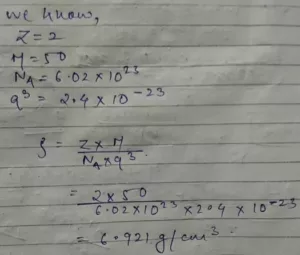Solid State Important Questions with Answers

14. An element crystallizes in a f.c.c. lattice with a cell edge of 400 pm. The density of the unit cell is 7 g cm-3. How many atoms are present in 280 g of the element?
Ans 14.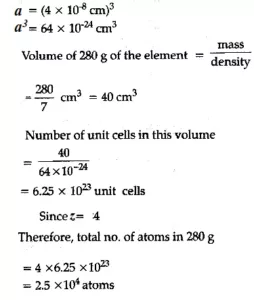15. Calculate the number of unit cells in 8.1 g of aluminium if it crystallizes in a face-centered Cubic (f.c.c) structure. (Atomic mass of Al =27 g mol).
Ans 15.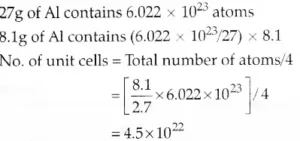Solid State Important Questions with Answers